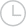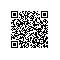# 快速比较数组-问答-阿里云开发者社区-阿里云

## 快速比较数组保持可爱mmm2020-02-09 13:53:01141

var myArray1 : [String] = ["1","2","3","4","5"] var myArray2 : [String] = ["1","2","3","4","5"]

// 1) Comparing 2 simple arrays

if(myArray1 == myArray2) { println("Equality") } else { println("Equality no") } // -> prints equality -> thanks god

// 2) comparing to a "copy" of an array

// swift copies arrays when passed as parameters (as per doc) func arrayTest(anArray: [String]) -> Bool { return anArray == myArray1 }

println("Array test 1 is (arrayTest(myArray1))") println("Array test 2 is (arrayTest(myArray2))") // equality works for both

myArray2.append("test") println("Array test 2 is (arrayTest(myArray2))") // false (obviously)

myArray2.removeAtIndex(5) println("Array test 2 is (arrayTest(myArray2))") // true 苹果表示，阵列拷贝背后存在优化。看起来有时（并非总是）结构实际上是否被复制。

1）==遍历所有数组以执行基于元素的比较吗？（看起来像）->那么在非常大的阵列上的性能/内存使用情况如何？

2）我们确定如果所有元素都相等，==会返回true吗？我对Java字符串的==记忆犹新

3）有没有一种方法可以检查myArray1和myArray2在技术上是否使用相同的“内存位置” /指针/等？我正在了解优化的工作原理和潜在的警告。

Java Swift取消 提交回答

• 保持可爱mmm
2020-02-09 13:53:15

您应该对以下内容有些紧张==：

struct NeverEqual: Equatable { } func ==(lhs: NeverEqual, rhs: NeverEqual)->Bool { return false } let x = [NeverEqual()] var y = x x == y // this returns true

[NeverEqual()] == [NeverEqual()] // false x == [NeverEqual()] // false

let z = [NeverEqual()] x == z // false

x == y // true

y = NeverEqual() x == y // now false 为什么？Swift数组不符合Equatable，但它们确实有一个==运算符，在标准库中定义为：

func == (lhs: [T], rhs: [T]) -> Bool 此运算符在lhs和中的元素上循环rhs，比较每个位置的值。它并没有做比较按位-它调用的==每个元素对运营商。这意味着，如果您==为元素编写自定义，它将被调用。

但是它包含一个优化-如果两个数组的基础缓冲区相同，就不会打扰，它只会返回true（它们包含相同的元素，当然它们是相等的！）。

这个问题完全是NeverEqual平等运算符的错。平等应该是可传递的，对称的和反身的，而这一平等不是反身的（x == x是错误的）。但是它仍然可能使您无意识。

Swift数组是写时复制的-因此，当您编写var x = y它时，它实际上并不能复制该数组，而只是将其x存储缓冲区指针指向y。只有在稍后更改x或时y，它才会创建缓冲区的副本，以使未更改的变量不受影响。这对于数组的行为类似于值类型，但仍然具有高性能至关重要。

在早期的Swift版本中，您实际上可以调用===数组（同样在早期的版本中，变异行为也有所不同，如果您进行了mutation x，y即使已声明为，也会改变let-吓坏了人们，所以他们改变了它） 。

您可以===使用以下技巧重现on数组的旧行为（除了依赖于戳和探查外，不依赖于实现，这完全依赖于实现）技巧：

let a = [1,2,3] var b = a

a.withUnsafeBufferPointer { outer in b.withUnsafeBufferPointer { inner in println(inner.baseAddress == outer.baseAddress) } }

0 0使用钉钉扫一扫加入圈子
+ 订阅